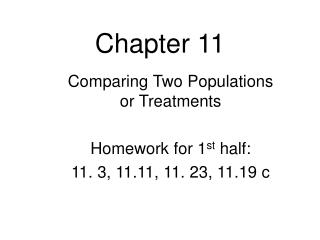DownloadDownload PresentationChapter 11

# Chapter 11

Télécharger la présentation## Chapter 11

- - - - - - - - - - - - - - - - - - - - - - - - - - - E N D - - - - - - - - - - - - - - - - - - - - - - - - - - -
##### Presentation Transcript

1. Chapter 11 Comparing Two Populations or Treatments Homework for 1st half: 11. 3, 11.11, 11. 23, 11.19 c

2. A sampling method is independent when the individuals selected for one sample does not dictate which individuals are to be in a second sample. A sampling method is dependent when the individuals selected to be in one sample are used to determine the individuals to be in the second sample. Dependent samples are often referred to as matched pairs samples.

3. EXAMPLE Independent versus Dependent Sampling For each of the following, determine whether the sampling method is independent or dependent. (a) A researcher wants to know whether a particular diet program was effective, so 20 individuals were randomly chosen and their weights were recorded before the program and after the program. (b) A researcher wishes to determine the effects of alcohol on reaction time. 50 people were randomly put into two groups of 25. Group 1 is given 2 drinks of alcohol, and Group 2 is given 2 drinks of alcohol-tasting placebo. Thirty minutes after the drinks, the subjects are given a test to determine reaction time.

4. Hypothesis Tests Comparing Two Means: Independent sampling Large size sample techniques allow us to test the null hypothesis H0: m1 - m2 = hypothesized value against one of the usual alternate hypotheses using the statistic

5. If n1 and n2 are both large or if the population distributions are normal and when the two random samples are independently selected, the standardized variable Has approximately a t distribution with Two-Sample t Test for Comparing Two Population Means: Independent Samples

6. Two-Sample t Test for Comparing Two Population Means If you use the classical method, this formula can be used to find the df, or you may use the conservative method of using the smallest ‘n-1’. We will only focus on the p-value method in this course for 2-sample tests.

7. Independent Samples: P-Value Method (Minitab will also give you the same results.) Once the assumptions have been confirmed (independent random samples, normally distributed), use these steps: • Stat, Tests, 2-SampZTest (if σ is Known) • Stat, Tests, 2-SampTTest (if σ is unknown) • Choose Data or Stats • Freq 1 and Freq 2 are always equal to 1 • Pooled “No” [To use ‘pooled’ we must show that the standard deviations are the same using a different test. In this course, we will always use the more conservative method: “Pooled: No”.] • Calculate • Compare p to α. If p < α, reject the null.

8. Hypothesis Test Brand A & B In an attempt to determine if two competing brands of cold medicine contain, on the average, the same amount of acetaminophen, twelve different tablets from each of the two competing brands were randomly selected and tested for the amount of acetaminophen each contains. The results (in milligrams) follow. Use a significance level of 0.01. Brand ABrand B 517, 495, 503, 491 493, 508, 513, 521 503, 493, 505, 495 541, 533, 500, 515 498, 481, 499, 494 536, 498, 515, 515 State and perform an appropriate hypothesis test.

9. Hypothesis Test Brand A & B m1 = the mean amount of acetaminophen in cold tablet brand A m2 = the mean amount of acetaminophen in cold tablet brand B H0:m1 = m2 (m1 - m2 = 0) Ha:m1m2(m1 - m20) Significance level:a = 0.01

10. Hypothesis Test Example Assumptions:The samples were selected independently and randomly. Since the samples are not large, we need to be able to assume that the populations (of amounts of acetaminophen are both normally distributed.

11. Hypothesis Test Example Assumptions (continued): As we can see from the normality plots and the boxplots, the assumption that the underlying distributions are normally distributed appears to be quite reasonable.

12. Hypothesis Test Brand A & B Solution Stat->Tests, 2-SampTTest Data, enter appropriate lists, Freq = 1 Select alternative hypothesis Pooled: “No” Calculate

13. Conclusion: Since P-value = 0.002 < 0.01 = a, H0 is rejected. The data provides strong evidence that the mean amount of acetaminophen is not the same for both brands. Specifically, there is strong evidence that the average amount per tablet for brand A is less than that for brand B.

14. EXAMPLE Comparing Two Means: Independent Sampling A researcher wanted to know whether “state” quarters had a weight that is more than “traditional” quarters. He randomly selected 18 “state” quarters and 16 “traditional” quarters, weighed each of them and obtained the following data.